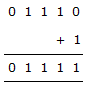# Electronics and Communication Engineering - Digital Electronics

41.

As applied to a flip flop the word edge triggered's means

 A. flip flop can change state when clock transition occurs B. flip flop can change state when clock signal goes from LOW to HIGH only C. flip flop can change state when clock signal goes from HIGH to LOW only D. none of the above

Explanation:

Edge triggering means the instant when clock transition occurs.

42.

A NOR gate is a combination of

 A. OR gate and AND gate B. AND gate and NOT gate C. OR gate and NOT gate D. two NOT gates

Explanation:

OR and NOT = NOR.

43.

The 2's complement representation of - 17 is

 A. 01110 B. 01111 C. 11110 D. 10001

Explanation:

(17)10 = (10001)2 = (-17)10 = 1's complement of (17)2 + 1the MSB is 0, hence (-17)2 cannot represented in 2's complement representation with 5 bit, therefore (-17) in 2's complement is simply (10001)2 = (17)10 .

44.

TTL inverter has

 A. one input B. two inputs C. one or two inputs D. three inputs

Explanation:

Data input and control input.

45.

4 bit ripple counter and 4 bit synchronous counter are made using flip-flop having a propagation delay of 10 ns each. If the worst case delay in the ripple counter and the synchronous counter be R and S respectively, then

 A. R = 10 ns, S = 40 ns B. R = 40 ns, S = 10 ns C. R = 10 ns, S = 30 ns D. R = 30 ns, S = 10 ns